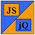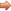# JavaScript & jQuery Tutorials

JS HomeJS ReferenceAssignment

## Assignment operators

Used for assignment from right to left.

### Description

Assignment operators allow us to assigns a value to a left operand based on the value of a right operand.

### Operators

Assignment Operator Description Example Result
`=`Equality `var a = 5, b = 10;`
`b = a;`
b = 5
`+=`Shorthand Addition `var a = 5, b = 10;`
`b += a;`
b = 15
`-=`Shorthand Subtraction `var a = 5, b = 10;`
`b -= a;`
b = 5
`*=`Shorthand Multiplication `var a = 5, b = 10;`
`b *= a;`
b = 50
`/=`Shorthand Division `var a = 5, b = 10;`
`b /= a;`
b = 2
`&=`Shorthand Bitwise AND `var a = 5, b = 10;`
`b &= a;`
b = 0
`|=`Shorthand Bitwise OR `var a = 5, b = 10;`
`b |= a;`
b = 15
`^=`Shorthand Bitwise XOR `var a = 5, b = 10;`
`b ^= a;`
b = 15
`<<=`Shorthand Left Shift `var a = 111111, b = 1010;`
`b <<= a;`
b = 129280
`>>=`Shorthand Sign-Propagating Right Shift `var a = 111111, b = 1010;`
`b >>= a;`
b = 7
`>>>=`Shorthand Zero-Fill Right Shift `var a = 111111, b = 1010;`
`b >>>= a;`
b = 7

## Related Tutorials

JavaScript Basic Tutorials - Lesson 5 - Basic Maths Functions